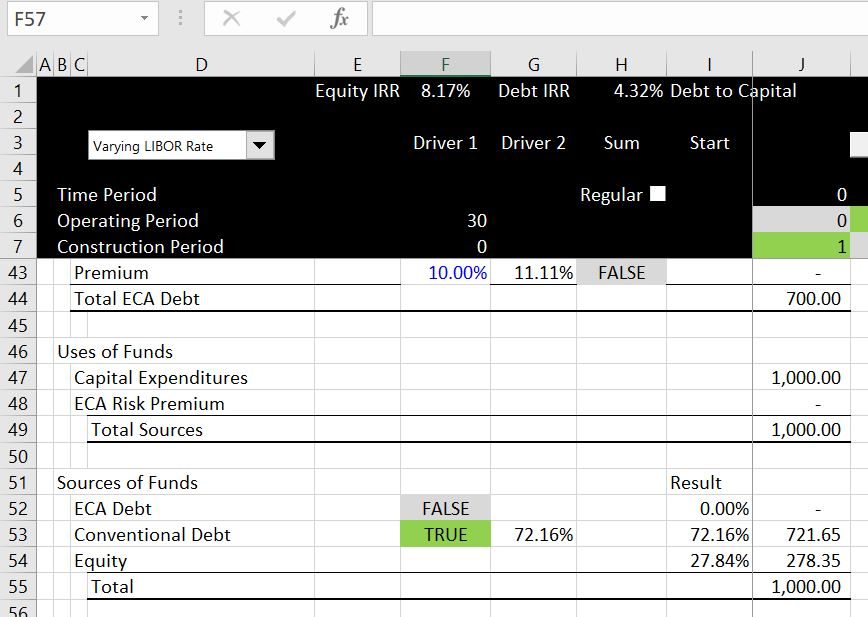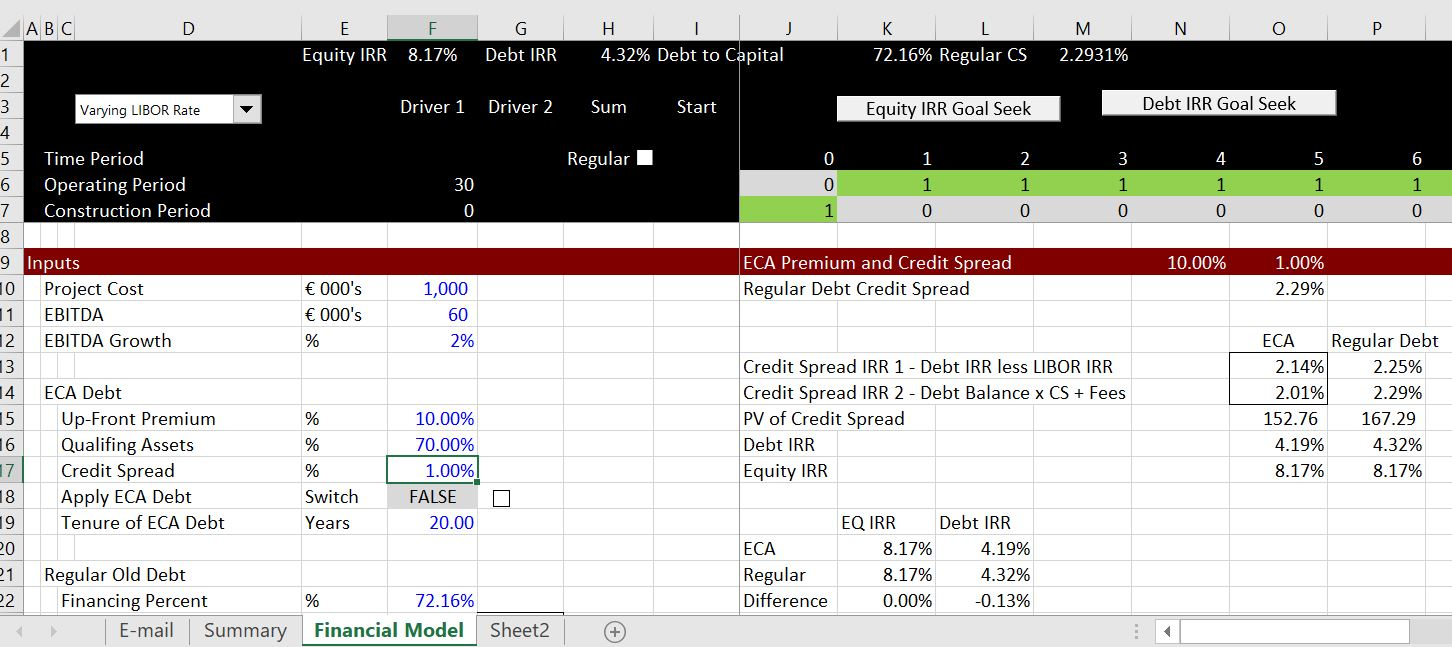# ECA Financing, Premium and Debt IRR

This page demonstrates modelling issues associated with Export Credit Agency Financing and how to measure the effective credit spread that includes a large up front premium or fee.  Different methods are presented for measuring the effective ECA credit spread including subtracting the LIBOR (or Euribor) IRR from the debt IRR and measuring the IRR on the Credit Spread and the up front premium.  I suggest that a good test is constructing a simple model with ECA and non ECA debt.  This can be used to prove what method really works instead of going around in circles.  I further suggest that backing into the credit spread with non-ECA debt from a goal seek on the equity IRR is a good method.

As with other pages, you can download the example spreadsheet below to illustrate the proof.  You can get the file by clicking on the button below.  The first file has blanks where you can try and work through some of the issues by yourself.  The second file is a completed file that you can work with in conjunction with the video below.

maxbutton id=493a]

If you look hard you can find the credit spread for the regular debt in F23.  This credit spread is used for non-ECA debt.  This is from a goal seek that makes the equity IRR equal to zero.  The table beginning in cell J13 shows results of the analysis. A goal seek is used to make the difference between regular debt and the ECA debt to be zero.  When this is done, the Credit Spread using the Debt IRR minus the Libor IRR (the 2.14%) is close to the theoretical credit spread (the 2.16% derived from the goal seek) but it is not exact.  The inexactness is driven by the fact that you cannot really just add up IRR’s when interest rates are changing. The method where the  IRR is computed separately on the Credit spread (the 2.01%) is lower then the correct amount.  The lower IRR comes from the fact that the IRR includes re-investment assumptions and when you exclude the LIBOR from the IRR.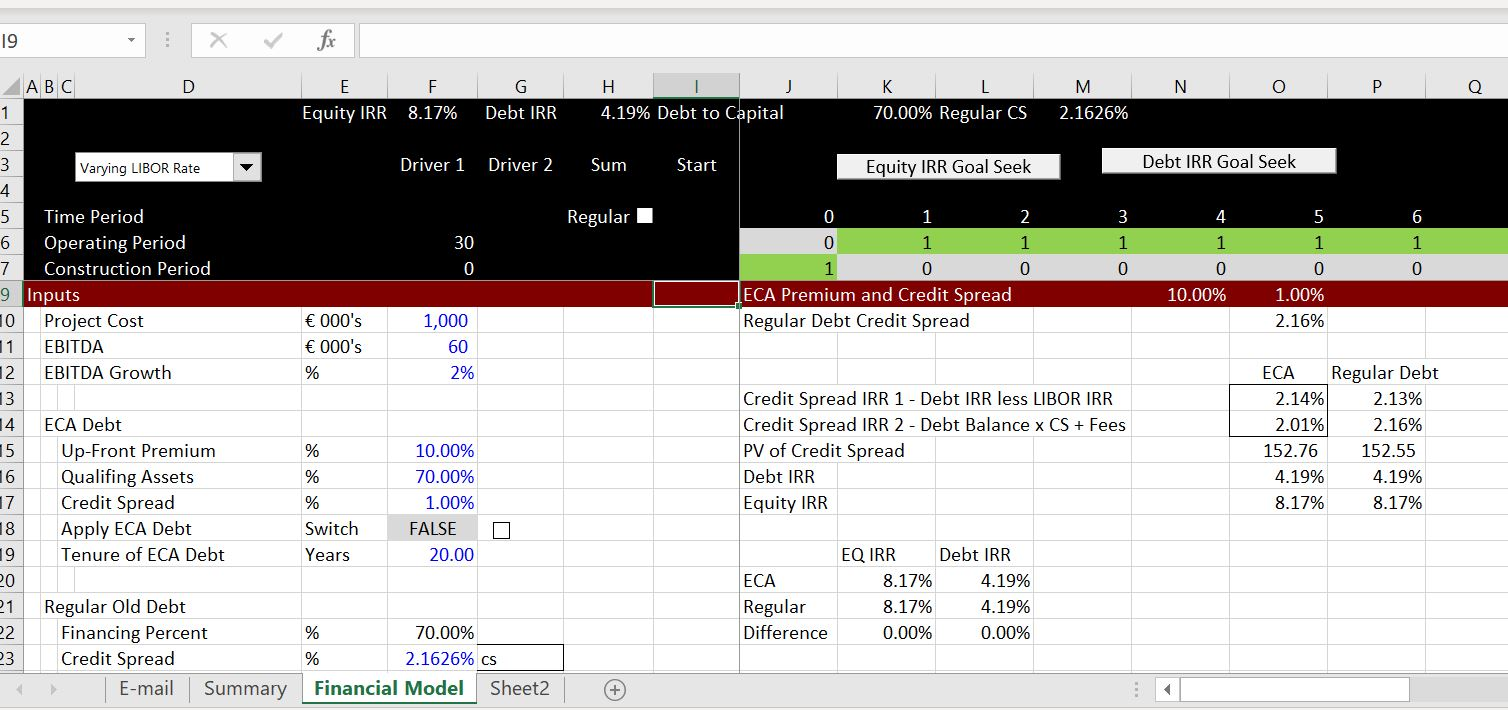The two screenshots below illustrate that when the interest rate is constant, you can add the IRR’s together.  But when the interest rates change, you cannot add the IRR’s.  In the first screenshot, there are two debt issues that each have a 5% interest rate.  You can add the two together and get the IRR of 10%.  In the second example, there are two debt issues, but the first debt issue has a changing rate.  In this case the addition of the IRR’s does not produce the correct IRR from adding the cash flows and then computing the IRR’s.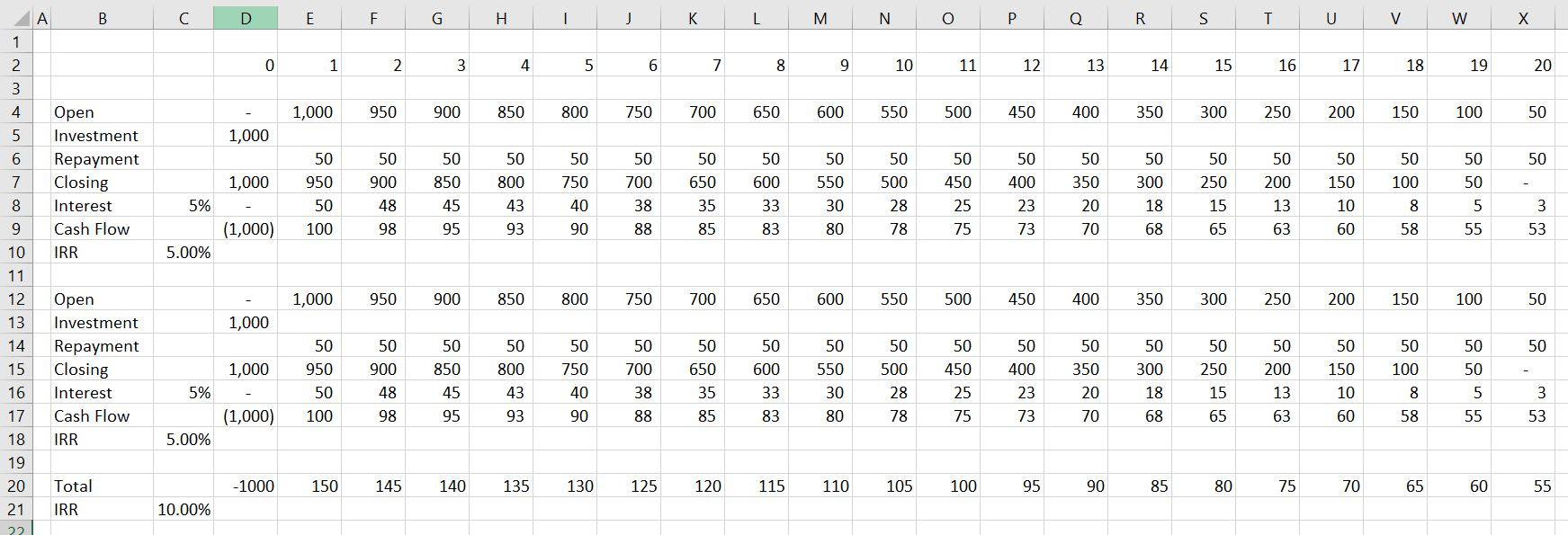The next couple of screenshots illustrate how the ECA works.  If you have regular old debt and multiple debt issues, you can just divide up the debt to compute the debt draws during the availability period.  I the premium is 10%, then the ECA debt can be increased by 1/(10%-1) so the debt covers the total debt (ECA debt = (ECA Assets + 1) * 10%))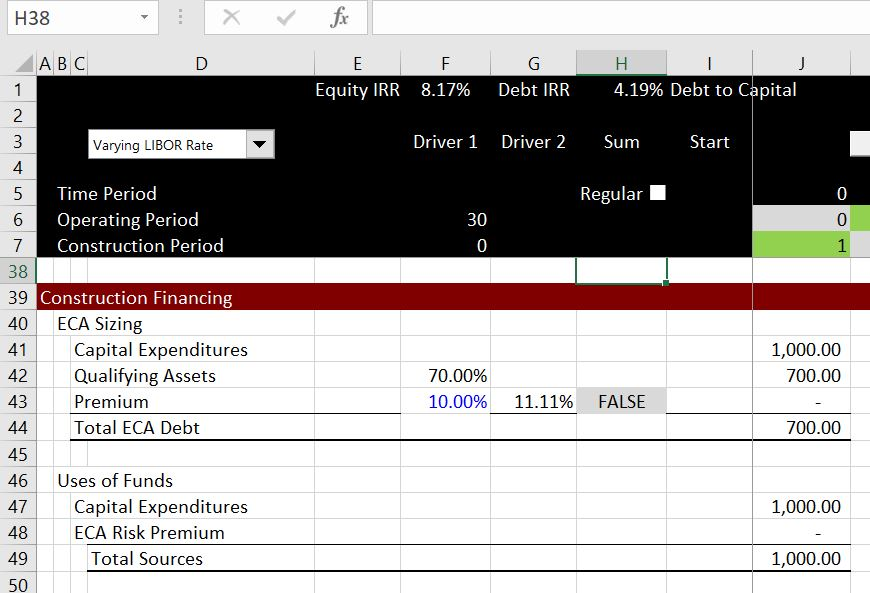In the screenshot below, ECA financing is illustrated. In this case the loan covers the assets that qualify for the ECA loan and also the up-front premium.